GeeksforGeeks App
Open AppBrowser
Continue

# Create Error Bars in Plotly – Python

Plotly is a Python library that is used to design graphs, especially interactive graphs. It can plot various graphs and charts like histogram, barplot, boxplot, spreadplot, and many more. It is mainly used in data analysis as well as financial analysis. plotly is an interactive visualization library.

## Error Bars in Plotly

For functions representing 2D data points such as px.scatter, px.line, px.bar, etc., error bars are given as a column name which is the value of the error_x (for the error on x position) and error_y (for the error on y position). Error bars are the graphical presentation alternation of data and used on graphs to imply the error or uncertainty in a reported capacity.

Example 1: In this example, we will plot a simple error plot using tips() data set.

## Python3

 `import` `plotly.express as px`` ` ` ` `df ``=` `px.data.tips()``df[``"error"``] ``=` `df[``"total_bill"``]``/``100`` ` `fig ``=` `px.scatter(df, x``=``"total_bill"``, y``=``"day"``, color``=``"sex"``,``                 ``error_x``=``"error"``, error_y``=``"error"``)``fig.show()`

Output: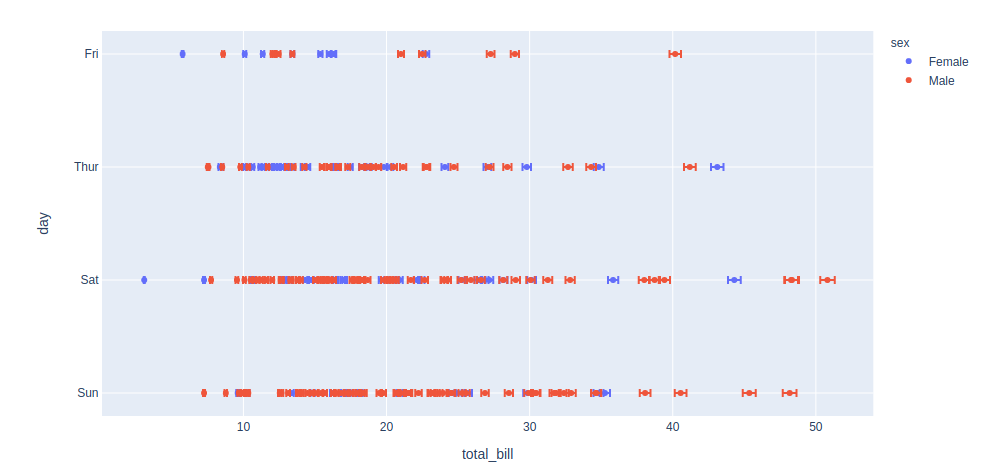Example 2:

## Python3

 `import` `plotly.express as px`` ` `df ``=` `px.data.tips()``df[``"e"``] ``=` `df[``"total_bill"``]``/``100`` ` `fig ``=` `px.bar(df, x``=``"total_bill"``, y``=``"day"``, color``=``"sex"``,``             ``error_x``=``"e"``, error_y``=``"e"``)``fig.show()`

Output: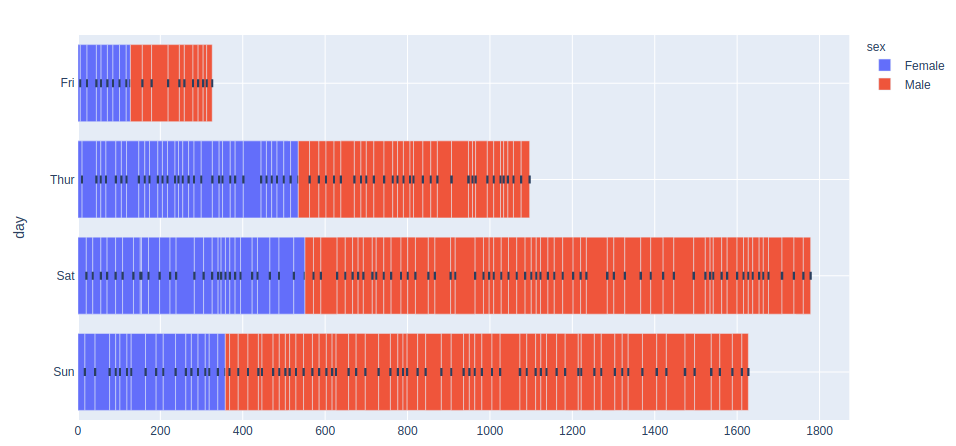The above example my seems something meshed up, but once you zoom it you’ll understand the graph more accurately.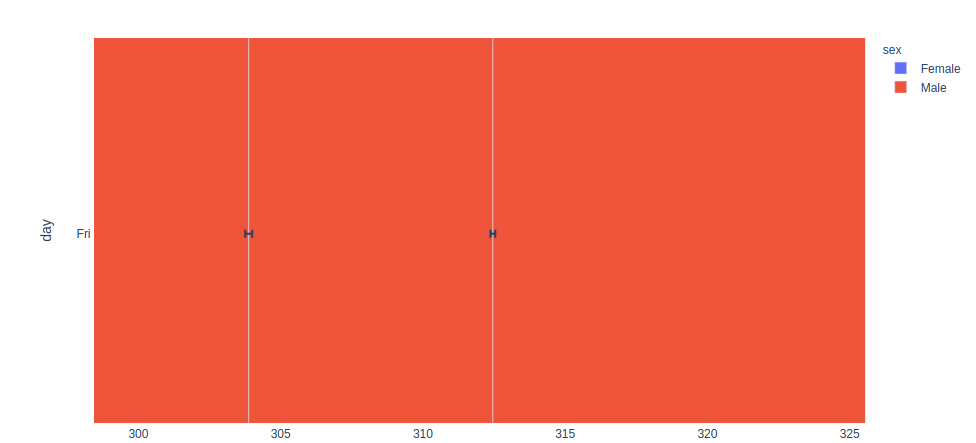Example 3: In this example, we will see Asymmetric Error Bars, Asymmetric errors arise when there is a non-linear dependence of a result.

## Python3

 `import` `plotly.express as px`` ` ` ` `df ``=` `px.data.tips()``df[``"error"``] ``=` `df[``"total_bill"``]``/``100``df[``"W_error"``] ``=` `df[``"total_bill"``] ``-` `df[``"tip"``] `` ` `fig ``=` `px.scatter(df, x``=``"total_bill"``, y``=``"day"``, color``=``"sex"``,``                 ``error_x``=``"error"``, error_y``=``"W_error"``)``fig.show()`

Output: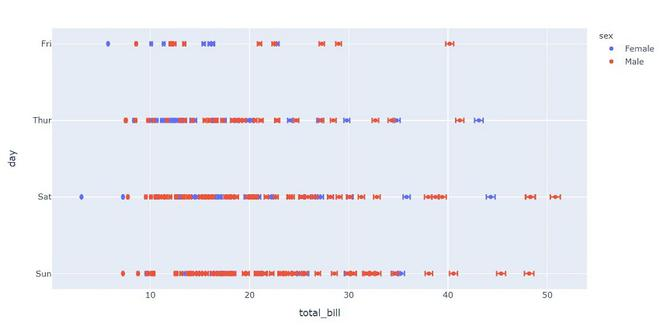Example 4: In this example, we will see Symmetric Error Bars, Symmetric mean absolute percentage error is an accuracy measure based on percentage errors.

## Python3

 `import` `plotly.graph_objects as go``x_data ``=` `[``1``, ``2``, ``3``, ``4``]``y_data ``=` `[``3``, ``5``, ``2``, ``6``]`` ` `fig ``=` `go.Figure(data ``=` `go.Scatter(``        ``x``=` `x_data,``        ``y``=` `y_data,``        ``error_y ``=` `dict``(``             ` `              ``# value of error bar given in data coordinates``            ``type` `=``'data'``,``            ``array ``=` `[``1``, ``2``, ``3``,``4``],``            ``visible ``=` `True``)``    ``))``fig.show()`

Output: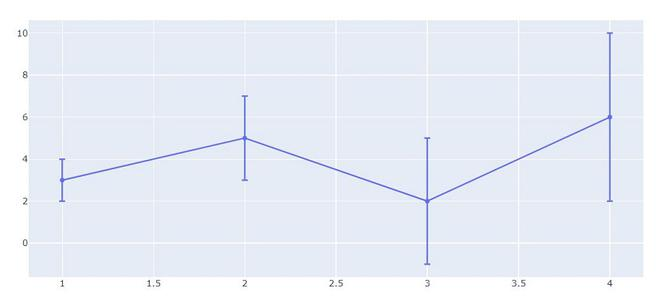Example 5: In this example, we will see how to coloring and styling the error bar using their attributes.

## Python3

 `import` `plotly.express as px``import` `plotly.graph_objects as go``import` `numpy as np`` ` `X ``=` `np.linspace(``-``1``, ``1``, ``100``)``Y ``=` `np.sinc(X)`` ` `x ``=` `[``-``0.89``, ``-``0.24``, ``-``0.0``, ``0.41``, ``0.89``, ]``y ``=` `[``0.36``, ``0.75``, ``1.03``, ``0.65``, ``0.28``, ]`` ` `fig ``=` `go.Figure()``fig.add_trace(go.Scatter(``    ``x``=``X, y``=``Y,``    ``name``=``'error bar'``))`` ` `fig.add_trace(go.Scatter(``    ``x``=``x, y``=``y,``    ``mode``=``'markers'``,``    ``name``=``'measured'``,``    ``error_y``=``dict``(``        ``type``=``'constant'``,``        ``value``=``0.1``,``        ``color``=``'green'``,``        ``thickness``=``1.5``,``        ``width``=``3``,``    ``),``    ``error_x``=``dict``(``        ``type``=``'constant'``,``        ``value``=``0.2``,``        ``color``=``'blue'``,``        ``thickness``=``1.5``,``        ``width``=``3``,``    ``),``    ``marker``=``dict``(color``=``'green'``, size``=``8``)``))`` ` `fig.show()`

Output: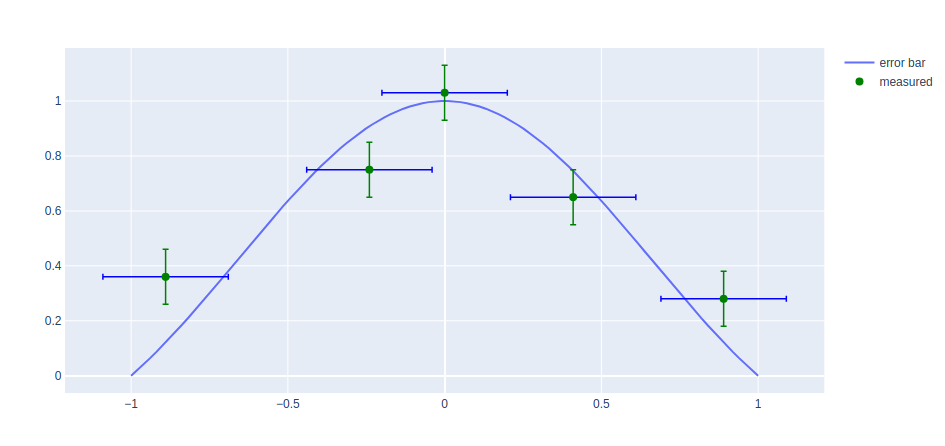My Personal Notes arrow_drop_up